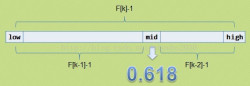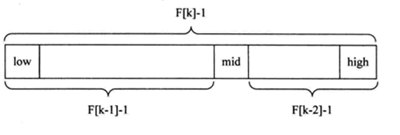• import java.util.Arrays; /** * @description: * @Author: muyi * @CreateDate: 2020/12/2 12:00 */ public class FibonacciSearch { /** * 斐波那契数组的长度 */ private final static int FIB
package com.itheima.security.springboot.algorithm.search;

import java.util.Arrays;

/**
* @description:
* @Author: muyi
* @CreateDate: 2020/12/2 12:00
*/
public class FibonacciSearch {

/**
* 斐波那契数组的长度
*/
private final static int FIB_ARRAY_LENGTH = 10;
/**
* 斐波那契数组的最小长度
*/
private final static int FIB_ARRAY_MIN_LENGTH = 3;

public static void main(String[] args) {
System.out.println(Arrays.toString(getFibArray()));
int[] array = {1, 5, 15, 22, 25, 31, 39, 42, 47, 49, 59, 68, 88, 88, 88, 88, 88};
System.out.println(array.length);
System.out.println(fibonacciSearch(array, 47));
System.out.println(recurseFibonacciSearch(array, getFibArray(), 47, 0, array.length - 1, 7));

}

/**
* 获取斐波那契数组
*
* @Author muyi
* @Date 12:07 2020/12/2
*/
private static int[] getFibArray() {
int[] fibArray = new int[FIB_ARRAY_LENGTH];
if (FIB_ARRAY_LENGTH < FIB_ARRAY_MIN_LENGTH) {
throw new IllegalArgumentException("斐波那契数列的最小长度为3");
}
fibArray = 1;
fibArray = 1;
for (int i = 2; i < FIB_ARRAY_LENGTH; i++) {
fibArray[i] = fibArray[i - 1] + fibArray[i - 2];
}
return fibArray;
}

/**
* 斐波那契查找（非递归）
*
* @param array 待查找的数组
* @param key   查找的关键字
* @return int 关键字在数组的位置，未查找到，返回-1
* @Author muyi
* @Date 12:08 2020/12/2
*/
public static int fibonacciSearch(int[] array, int key) {
if (array == null || array.length == 0) {
return -1;
}
int length = array.length;
// 制造一个长度为10的斐波数列,要特别明确：这里面的数据是索引值！！！！
int[] fb = getFibArray();
int k = 0;
// 找出数组的长度在斐波数列（减1）中的位置，将决定如何拆分！
while (length > fb[k] - 1) {
// 此时K的值为斐波那契数列中大于【数组长度的最小值】所在的索引值
k++;
}
// 构造一个长度为fb[k] - 1的新数列，构造查询的中间数组
int[] temp = Arrays.copyOf(array, fb[k]);
// 获取数组最后一个位置的元素值，用该值对新数列length以后的元素进行填充
int arrayLastValue = array[length - 1];
for (int i = length; i < temp.length; i++) {
temp[i] = arrayLastValue;
}

// 刚开始是在整个中间数组中进行茶渣，即对查询数组进行黄金分割
int low = 0;
int high = array.length - 1;
while (low <= high) {
/**
* 从 temp 数组中找黄金分割点，
* 黄金分隔点即是斐波那契数组的前一索引位置的数字
* 斐波那契的特点：f[k] = f[k-1] + f[k-2] ===》 f[k]-1 = f[k-1]-1 + f[k-2]-1 +1
* 黄金分割点的值为 f[k-1] -1,正常黄金分割点的值应该是f[k-1],但这里代表的是数组的索引，从0开始，故这里需要对其进行-1操作
*                         low是作为获取黄金分隔点的基准值
*/
int middle = low + fb[k - 1] - 1;
// 如果黄金分隔点的数大于关键字，那继续从黄金分割点之前查找
if (temp[middle] > key) {
high = middle - 1;
// 前面的元素个数是 f[k-1] 个
k--;
}
// 如果小于关键字，那继续从黄金分割点之后查找
else if (temp[middle] < key) {
low = middle + 1;
// 后面的元素个数是 f[k-2] 个
k -= 2;
} else {
// 若相等则说明mid即为查找到的位置
if (middle <= high) {
return middle;
}
// middle的值已经大于high,进入扩展数组的填充部分,即最后一个数就是要查找的数。
else {
return high;
}
}
}
return -1;
}

/**
* 斐波那契查找（递归方式）
*
* @param array    待查找的数组
* @param fibArray 斐波那契数组
* @param key      关键字
* @param low      低索引值
* @param high     高索引值
* @param index    索引值，即查找数组最大长度所对应的斐波那契数组中数据的索引值
* @return int 关键字在数组的位置，未查找到，返回-1
* @Author muyi
* @Date 14:32 2020/12/2
*/
public static int recurseFibonacciSearch(int[] array, int[] fibArray, int key, int low, int high, int index) {
/**
*  key < array[low]：小于最小值
*  key > array[high]：大于最大值
*  low > high：遍历整个数组均未找到元素，退出
*/
if (array == null || array.length == 0 || key < array[low] || key > array[high] || low > high) {
return -1;
}
int middle = low + fibArray[index - 1] - 1;
if (key < array[middle]) {
// 如果小于关键字，在黄金分隔点之前查找
return recurseFibonacciSearch(array, fibArray, key, low, middle - 1, index - 1);
} else if (key > array[middle]) {
// 如果大于关键字，在黄金分割点之后查找
return recurseFibonacciSearch(array, fibArray, key, middle + 1, high, index - 2);
} else {
// 若相等则说明 middle 即为查找到的位置
if (middle <= high) {
return middle;
// middle的值已经大于high,进入扩展数组的填充部分,即最后一个数就是要查找的数。
} else {
return high;
}
}
}
}

展开全文算法
• 一、什么是斐波那契查找 斐波那契搜索就是在二分查找的基础上根据斐波那契数列进行分割的。在斐波那契数列找一个等于略大于查找表中元素个数的数F[n]， 将原查找表扩展为长度为F[n](如果要补充元素，则补充重复最后...

一、什么是斐波那契查找

斐波那契搜索就是在二分查找的基础上根据斐波那契数列进行分割的。在斐波那契数列找一个等于略大于查找表中元素个数的数F[n]， 将原查找表扩展为长度为F[n](如果要补充元素，则补充重复最后一个元素，直到满足F[n]个元素)，完成后进行斐波那契分割，即F[n]个元素分割为前半部分F[n-1]个元素，后半部分F[n-2]个元素，找出要查找的元素在那一部分并递归，直到找到。查找表是顺序存储的有序表

三、查找过程

1. 斐波那契查找原理与前两种相似，仅仅改变了中间结点（mid）的位置，mid不再是中间或插值得到，而是位于黄金分割点附近，即mid=low+F(k-1)-1（F代表斐波那契数列），如下图所示2. 对F(k-1)-1的理解：
• 由斐波那契数列 F[k]=F[k-1]+F[k-2] 的性质，可以得到 （F[k]-1）=（F[k-1]-1）+（F[k-2]-1）+1 。该式说明：只要顺序表的长度为F[k]-1，则可以将该表分成长度为F[k-1]-1和F[k-2]-1的两段，即如上图所示。从而中间位置为mid=low+F(k-1)-1

• 类似的，每一子段也可以用相同的方式分割

• 顺序表长度n不一定刚好等于F[k]-1，所以需要将原来的顺序表长度n增加至F[k]-1。这里的k值只要能使得F[k]-1恰好大于或等于n即可，由以下代码得到,顺序表长度增加后，新增的位置（从n+1到F[k]-1位置），都赋为n位置的值即可。

四、代码实现

import java.util.Arrays;

public class FibonacciSearch {
public static void main(String[] args) {
int[] array = {1,8,10,89,1000,2000};
System.out.println("index="+fibonacciSearch(array,-1));
}

/**
* @return 斐波那契数列
*/
public static int[] fibonacciSequence(int maxSize) {
int[] f = new int[maxSize];
f = 1;
f = 1;
for (int i = 2; i < maxSize; i++) {
f[i] = f[i - 1] + f[i - 2];
}
return f;
}

/**
* 斐波那契查找
* @param arrays 传入数组
* @param value 待搜索的值
* @return 下标
*/
public static int fibonacciSearch(int[] arrays, int value) {
int left = 0;
int right = arrays.length - 1;
int mid = 0;
//存放斐波那契数列
int[] fibArray = fibonacciSequence(10);
//表示斐波那契分割数值的下标
int fibIndex = 0;
//获取到斐波那契分割数值的下标
while (right > fibArray[fibIndex] - 1) {
fibIndex++;
}

//fibArray[fibIndex]的值可能大于a的长度，因此需要构建一个新数组，不足的部分会使用0填充
int[] temp = Arrays.copyOf(arrays, fibArray[fibIndex]);

//将新填充的内容替换为最后的数
//例：temp = {1,3,4,6,9,11,0,0} => {1,3,4,6,9,11,11,11}
for (int i = right + 1; i < temp.length; i++) {
temp[i] = arrays[right];
}
//使用while来循环处理，找到value，前提是左指针在右指针前边
while (left <= right) {
mid = left + fibArray[fibIndex - 1] - 1;
//当查找的值小于当前值时应该向数组的前边遍历
if (value < temp[mid]) {
right = mid - 1;
//斐波那契数向前移一位
fibIndex--;
}
//当查找的值小于当前值时应该向数组的后边遍历
else if (value > temp[mid]) {
left = mid + 1;
fibIndex -= 2;
} else {
if (mid <= right) {
return mid;
} else {
return right;
}
}
}
return -1;
}
}

展开全文数据结构 java 算法 leetcode
• 斐波那契查找Java斐波那契查找就是在二分查找的基础上根据斐波那契数列进行分割的。在斐波那契数列找一个等于略大于查找表中元素个数的数F[n]，将原查找表扩展为长度为Fn，完成后进行斐波那契分割，即F[n]个...

斐波那契查找（Java）

斐波那契查找就是在二分查找的基础上根据斐波那契数列进行分割的。在斐波那契数列找一个等于略大于查找表中元素个数的数F[n]，将原查找表扩展为长度为Fn，完成后进行斐波那契分割，即F[n]个元素分割为前半部分F[n-1]个元素，后半部分F[n-2]个元素，找出要查找的元素在那一部分并递归，直到找到。

重要公式：mid=low+F[k-1]-1
适用条件： 采用顺序存储结构的有序表
理解：mid在黄金分割点附近，
F(k-1)-1:是由斐波那契数列的“F[k]=F[k-1]+F[k-2]”,F[k]-1=(F[k-1]-1)+(F[k-2]-1)+1,则若顺序表的长度为：F[k]-1,则可分成F[k-1]-1和F[k-2]-1两段，中间位置就为：mid=low+F[k-1]-1代码如下

import java.util.Arrays;

public class Fibonaccisearch {
public static int maxsize=20;
public static void main(String[] args) {
int arr[]= {2,3,5,7,9,11};
int Index=fibonaccisearch(arr,5);
if(Index==-1) {
System.out.println("没有该元素");
}else {
System.out.println("斐波那契查找，该元素的下标为："+Index);
}
}
public static int fibonacci(int i){
if(i==0||i==1) {
return i;
}else {
return fibonacci(i-1)+fibonacci(i-2);
}
}
public static int fibonaccisearch(int []arr,int  key) {
int low=0;
int high=arr.length-1;
int k=0;
int mid=0;
int []f=new int[maxsize];
for(int i=0;i<f.length;i++) {
f[i]=fibonacci(i);
}
while(high>f[k]-1) {//获得斐波那契分割点
k++;
}
int[]temp=Arrays.copyOf(arr, f[k]);
for(int j=high+1;j<temp.length;j++) {
temp[j]=arr[high];
}
while(low<=high) {
mid=low+f[k-1]-1;
if(key<temp[mid]) {//向左边查找
high=mid-1;
k--;
}else if(key>temp[mid]) {
low=mid+1;
k=k-2;
}else {
if(mid<=high) {
return mid;
}else {
return high;
}
}
}
return -1;
}
}

运行结果
斐波那契查找，该元素的下标为：2

展开全文数据结构 算法 java
• package cn.mrlij.search; import java.util.Arrays; public class FibonacciSearch { public static int maxSize = 20; public static void main(String[] args) { int arr[] = {2,3,6,8,9,20};...
package cn.mrlij.search;

import java.util.Arrays;

public class FibonacciSearch {
public static int maxSize = 20;
public static void main(String[] args) {
int arr[] = {2,3,6,8,9,20};
System.out.println(fibSearch(arr,20));
}
//首先得到一个斐波那契数列，mid的值是low+F(k-1)-1
public static int[] fib(){
int f[] = new int[maxSize];
f = 1;
f = 1;
for (int i = 2; i < maxSize; i++) {
f[i] = f[i-1] + f[i-2];
}
return f;
}
//斐波那契查找算法
public static int fibSearch(int[] arr,int key){
int low = 0;
int high = arr.length -1;
int k = 0;//表示斐波那契分割的值
int mid = 0;//存放黄金分割点的值
int f[] = fib();//得到斐波那契数列
//找到k值
while (high>f[k] - 1){
k++;
}
//复制到新的数组
int temp[] = Arrays.copyOf(arr,f[k]);
//得到f[k]的值可能会大于a的长度,将新数组的多余的部分用数组中的最后一个数字填充
for (int i = high+1; i <temp.length ; i++) {
temp[i] = arr[high];
}
//使用while循环，找到key
while (low<=high){//满足这个条件就可以继续找
mid = low+f[k-1]-1;//找到的黄金分割点
if(key<temp[mid]){//查找的值小于该点的时候就钱后找
high = mid - 1;
k--;
}else if(key>temp[mid]){
low = mid + 1;
k-=2;
}else{
if(mid<=high){
return mid;
}else {
return high;
}
}
}
return -1;
}
}

展开全文斐波那契查找算法
• 什么是斐波那契查找 斐波那契数列，又称黄金分割数列，指的是这样一个数列：1、1、2、3、5、8、13、21、····，在数学上，斐波那契被递归方法如下定义：F(1)=1，F(2)=1，F(n)=f(n-1)+F(n-2) （n&gt;=2）。该...
• import java.util.Arrays; public class FibonacciSearch { public static int maxSize = 20; public static void main(String[] args) { int[] arr = {1, 8, 10, 89, 1000, 1234}; Syst...
• Java基础查找算法(4)——斐波那契查找 1.斐波那契查找简述 斐波那契查找也称黄金分割法，与二分查找和插值查找类似，只是mid的选取有所不同。 Java基础查找算法(2)——二分查找(折半查找) Java基础查找算法(3)——...斐波那契查找
• 二者与二分查找思想类似，只不过划分...斐波那契的中值点为: int mid = left + fibo[k - 1] - 1; 具体实现代码如下 插值 public static int insertResearch(int[] arr,int left,int right,int value) { if (ljava 开发语言 算法 数据结构
• } //编写斐波那契查找算法 public static int fabnacciSearch(int[] arr,int findVal) { int low=0; int high=arr.length-1; int k=0; //表示斐波那契分割数值得下标 int mid=0; int[] f=Fabonacci...数据结构 java 算法
• 斐波那契查找算法java版

千次阅读 2014-09-28 19:26:14
与二分查找相比，斐波那契查找算法的明显优点在于它只涉及加法和减法运算，而不用除法。因为除法比加减法要占去更多的机时，因此，斐波那契查找的平均性能要比折半查找好。 public class Fibonacci_Search ...
• 斐波那契查找算法：前提是一个有序数组。 斐波那契数列：1,1,2,3,5,8,13,21。。。。。。 主要思想：通过斐波那契数列找到数组黄金分割点附近的下标，即mid。 根据上图所示：假设有一个数组，数组里面的元素有F[k]-1...算法 数据结构 java
• package drug.search;...import java.util.Arrays; /** * @author Drug * @create 2020-04-29 16:10 */ public class FibonacciSearch { public static void main(String[] args) { int[] arr = {1,3...算法
• 斐波那契查找法类似与二分查找法，但是不同的是，是以黄金分割点来进行查找的，而斐波那契数列的相邻两项之比正好与黄金分割相似，所以可以利用斐波那契数列的值来计算。 在这个算法中，必须使用顺序数组，而且在...java 算法 数据结构
• 斐波那契搜索就是在二分查找的基础上根据斐波那契数列进行分割的。在斐波那契数列找一个等于略大于查找表中元素个数的数F[n]，将原查找表扩展为长度为F[n](如果要补充元素，则补充重复最后一个元素，直到满足F[n]个...
• 详细介绍了常见的4种查找算法，比如顺序查找/线性查找、二分查找/折半查找、插值查找斐波那契查找等，并且提供了相应的Java代码实现。java 数据结构 算法 查找 二分查找
• 二分查找 时间复杂度:O(logn) 算法的思路是： 1.区间为左闭右开[lo,hi) 2. 每次while循环只进行一次大小判断e<nums[mid]，使代码每次判断的时间常量更小。 public static int binSearch(int[] nums, int lo, int ...算法 数据结构 排序算法 快速排序
• 斐波那契查找—Java实现

千次阅读 2018-08-20 17:13:10
同样地，斐波那契查找也属于一种有序查找算法。 相对于折半查找，一般将待比较的key值与第mid=（low+high）/2位置的元素比较，比较结果分三种情况： 　1）相等，mid位置的元素即为所求 　2）&gt;，low=mid+1;...斐波那契查找
• 斐波那契查找

2020-08-21 06:42:43
2019-07-17 斐波那契数列，又称黄金分割数列，因数学家列昂纳多·斐波那契以兔子繁殖为例而引入，故又称为”兔子数列“，指的是这样一个数列：1、1、2、3、5、8、13、21、34、… ；...斐波那契查找就是在二查找
• 斐波那契查找原理与前两种相似，仅仅改变了中间结点（mid）的位置，mid不再是中间或插值得到，而是位于黄金分割点附近。数据结构 算法 java
• 一、斐波那契查找算法介绍 斐波那契数列（Fibonacci sequence），又称黄金分割数列、因数学家莱昂纳多·斐波那契（Leonardoda Fibonacci）以兔子繁殖为例子而引入，故又称为“兔子数列”，指的是这样一个数列：0、1...
• import java.util.Arrays; public class FibonacciSearch { public static int maxSize = 20; public static void main(String[] args) { int [] arr = {1,8, 10, 89, 1000, 1234}; System.out.println(...算法 数据结构 java
•    类似于二分法查找，它们的不同之处在于，斐波那契查找查找点的对半选择改进为黄金分割点选择，所以又叫做黄金比例查找法； 其中 searchVal代表需要查找的值，fid是斐波那契数列； 2、基础知识 2.1 斐波那契...java 算法
• 插值查找： 二分查找在确定中间值的位置的时候，使用的公式为： mid = (right + left) / 2 此公式可以转换为：mid = left + (right - left) / 2 上面的公式中 1/2 我们可以进行优化： 设要查找的值为 targetVal...java插值查找 斐波那契查找
• 1、什么是斐波那契数列？ 1、1、2、3、5、8、13、21、34…… 斐波那契数列又被成为黄金分割数列，因为 前一项/后一项越来越趋近于0.618 ...2、斐波那契查找斐波那契数列有什么联系？ 斐波那契查找原理与前两种相...数据结构 算法 java
• 2斐波那契数列 public static int fibonacci(int n){ if(n==1||n==2){ return 1; } return fibonacci(n-1)+fibonacci(n-2); } public static void main(String[] args) { int reslut=fibonacci(9); System.out...
• Java斐波那契查找算法 package myfirstprogram; import java.util.Arrays; public class Fibonacci_Search { //因为java没有斐波那契数列的引用方法，所以得构造数列 public static int[] fib(int n) { int[] ...数据结构 java 算法
• java语言实现二分查找 /* 二分查找法 */ public class BinarySearch { public static void main(String[] args) { int[] arr = {1,2,3,4,5,6,7,8}; int i = binarySearch1(arr,0,arr.length - 1, -1); System....java 算法 数据结构 二分法
• Fibonacci查找算法 package it.cast.Sort_Algorithm.Sort_Algorithm; import java.util.Arrays; public class FibonacciSort { public static void main(String[] args) { int[] array = new int[]{3,7,1,9,35...java 算法 数据结构...

斐波那契查找javajava 订阅